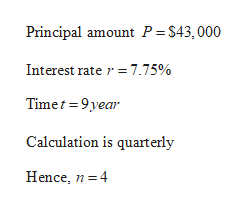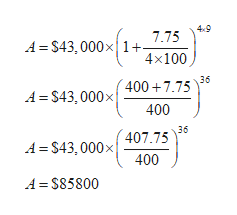# Find the accumulated amount A if the principal P is invested at the interest rate of r/year for t years. (Use a 365-day year. Round your answer to the nearest cent.)P = \$43,000, r = 7.75%, t = 9,compounded quarterly

Question

Find the accumulated amount A if the principal P is invested at the interest rate of r/year for t years. (Use a 365-day year. Round your answer to the nearest cent.)

P = \$43,000, r = 7.75%, t = 9,
compounded quarterly
check_circleExpert Solution
Step 1

Given values:help_outlineImage TranscriptionclosePrincipal amount P = \$43,000 Interest rate r7.75% Timet 9year Calculation is quarterly Hence, n4 fullscreen
Step 2

Formula for accumulated amount is:

Step 3

Putting the values in ab...help_outlineImage Transcriptionclose49 4=S43,000x1+ 7.75 4x100 A=S43,000x4007.75 400 36 A S43,000x 36 A=S43,000x 407.75 400 A = S85800 fullscreen

### Want to see the full answer?

See Solution

#### Want to see this answer and more?

Solutions are written by subject experts who are available 24/7. Questions are typically answered within 1 hour*

See Solution
*Response times may vary by subject and question
Tagged in

### Other